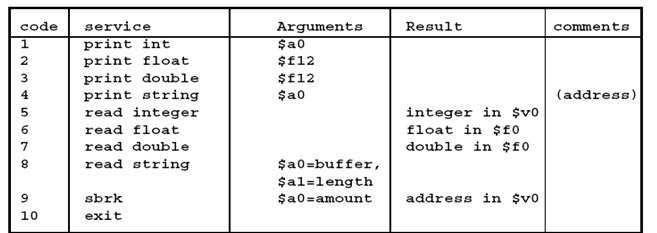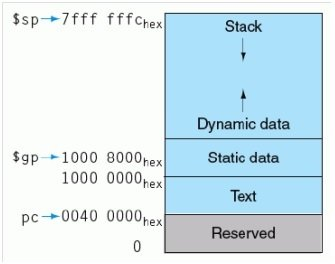# MIPS System Calls, Procedure Calls and Using the Stack

SYSTEM CALLS in MIPS: System call is used to communicate with the system for reading from keyboard or writing to the screen. A System call requires some parameter to be passed in a particular register and a request/function number (or service code) to be passed in register \$v0.

Steps for using System Calls:

1. Load service code into register \$v0. Codes (the values to be loaded in \$v0) and their respective functions are given in the table below.
2. Load arguments (if any) into registers such as \$a0, \$a1 according to the table.
3. Use syscall.
4. Results are returned in registers such as \$v0 according to the table.
5. All programs should terminate with \$v0 = 10 and syscall to Exit. This helps in running very long programs (which are run using the GO option in simulation menu in PCSPIM)### Exercise 1: Printing your Name using SYSCALL

```Use the following code to print your name on an output screen.

.data

mesg1:    .asciiz “my name is bilal malik“ # fill the blank with your name

.text

.globl main

main:

li   \$v0, 4        # Load immediate \$v0 with value 4

la   \$a0, mesg1    # \$a0 points to base address of string array mesg1

syscall

li   \$v0, 10       # prepare to exit

syscall           # Exit to OS```

Run this program by pressing GO (F5).  check the execution of your program and check how system calls are used to get input from user and display any thing on pcspim console.What do you see in the CONSOLE window? I see my name because I have printed “my name is bilal malik”

Now read the code and get understanding of followings line to get this code completely:

1. In the above code, what is the purpose of  \n?

\n is used for the purpose of starting a new line.

1. What is meant by asciiz (different from ascii)?

Both .asciiz and .ascii store a string in memory. However, .ascii does not null terminate the string while .asciiz null terminates it.

1. What does the first SYSCALL do (see table)?

The first syscall finds 4 in \$v0 and thus, it prints the string whose base address is found in the register \$a0.

1. What argument is contained in \$a0 before first SYSCALL

\$a0 contains the base address of the string to be printed on the syscall.

1. What is purpose of second SYSCALL (see table)

The second syscall finds 10 in \$v0 and thus, it exits the program.

### Exercise 2: Printing an Integer using SYSCALL

Modify the above program to print an integer (your roll number such as 15100900). Write your code below:

Code:

```.data

mesg2: .word 15100900

.text

.globl main

main:

li \$v0, 1     #Place 1 in \$v0 in order to print an integer

la \$t0, mesg2 #Put address of the memory location in \$t0

lw \$a0, 0(\$t0) #Load the integer from memory into \$a0

syscall       #syscall to print the integer

li \$v0, 10    #Prepare to exit by placing 10 in \$v0

syscall       #Exit to OS```

check the execution of your program

For printing an Integer, answer the following questions:

1. What should be the value in register a0 before you make the syscall: \$a0 should contain the integer to be printed.
2. What should be the value in register v0 before you make the syscall: \$v0 should have the value 1.

### Exercise 3: Sum of Integers using system calls using pcspim

Write a code that asks the user to enter a positive integer N and returns the Sum of all integers less than or equal to N. The sample code is given on the next page.  Note that, now you can run for N = 100, N= 200, N= 1000 by pressing GO, watching the console window, entering the value and hitting the Enter key.  What is the answer in each case?

For N=100, Sum: 5050, For N=200, Sum: 20100, For N=1000, Sum: 500500,

What happens if you give a very large N, say 100000? Can you explain why this happens? For a very large value of N like 100000, an exception occurs due to arithmetic overflow. For a large value of N, the corresponding sum is an even larger value and a time comes when the intermediate sum overflows into the signed bit of the register in which it is stored. Since positive signed numbers are being added, the sum itself cannot be negative and hence an exception occurs due to the arithmetic overflow into the signed bit. The instruction which is responsible is add \$t0, \$t0, \$v0

Since we have provided the code for Exercise 3 to you, the grading of this exercise will be done by testing your understanding of the given program through a viva. You are also required to show your .asm/.s code and its PSCPIM execution to the TA during the lab and get your handout marked.

Sample Code:

(Taken from the book: “MIPS Assembly Language Programming” by Robert L. Britton)

#######################################################################

# Cross References:

# v0: N, t0: Sum

#######################################################################

.data

Prompt:          .asciiz “\n  Please Input a value for N = (−1 to end): “

Result:           .asciiz “\n The sum of the integers from 1 to N is:  ”

Bye:                .asciiz “\n  **** Good bye – Have a good day****”

.text

.globl  main

main:

li                      \$v0, 4                         # system call code for print string

syscall                                                # print the prompt message

li                      \$v0, 5                         # system call code for Read Integer

syscall                                                # reads the value of N into \$v0

ble                   \$v0, \$zero, End        # branch to end if \$v0 <= 0

li                      \$t0, 0                          # clear register \$t0 to 0

Loop:

add                 \$t0, \$t0, \$v0               # sum of integers in register \$t0

addi                \$v0, \$v0, −1               # summing integers in reverse order

bne                 \$v0, \$zero, Loop       # branch to loop if \$v0 is != 0

li                      \$v0, 4                         # system call code for Print String

syscall                                                # print the string

li                      \$v0, 1                         # another system call to Print Integer

move              \$a0, \$t0                      # move value to be printed to \$a0

syscall                                                # print sum of integers

j                       main                           # go for next number

End:                li                      \$v0, 4                         # system call code for Print String

syscall                                                # print the string

li                      \$v0, 10                       # system call code for exit

syscall                                                # terminate the program & return control

### MIPS PROCEDURE CALLS

Exercise 1:

Let’s say we have a ‘check511’ function that returns 1 if the value passed to it is greater than 511 and 0 otherwise, as described in the following C++ program.  Let us convert this code to MIPS Assembly Language.  CIN and COUT can be decoded using SYSCALLs for reading and printing an integer.

C++ Code:

```int check511( int z );

int main ( )

{

int x, y;

cin>>x;

y = check511(x);

cout<<y;

}

//the function

int check511 ( int z )

{

int result;

if (z > 511)

{

result = 1;

}

else

{

result = 0;

}

return result;

}```

```For the MIPS Assembly Language, the template for the program that uses check511 procedure (function) is provided on the next page. In the main part of the code, each blank line stands for exactly one instruction. The purpose of each instruction is defined in terms of corresponding comments (which follow the # sign). The template shows how to call a procedure in terms of comments. In addition, it shows how to read from the keyboard and write the answer to the screen using SPIM system calls, again in terms of comments. You are required to fill in the blanks with the necessary MIPS Assembly Language instructions. Be sure to use the correct registers required for system and procedure calls as discussed in this lab earlier. The check511 procedure follows the label check511. You are required to develop the code for this procedure and write it down as well (in the space provided).

# Using a procedure in main

.text

.globl main

main:

# get input --- the cin part

addi    \$v0, \$zero, 5             # Use system call code 5 (read integer – See Table 1)

syscall                                    # Invoke system call (int is returned in \$v0)

add     \$a0, \$zero, \$v0         # put the input integer into \$a0 which is one of the

# argument registers for parameter passing

jal check511                          # call check511 procedure

# cout y  --  print output

add     \$a0, \$zero, \$v0         # put return value into \$a0

addi    \$v0, \$zero, 1             # Use system call 1 (print integer)

syscall                                    # Invoke system call

Exit:

addi    \$v0, \$zero, 10           # Use system call 10 (exit)

syscall                                    # Invoke system call

check511:

addi \$v0, \$zero, 0                 #Place 0 in the register \$v0

addi \$t0, \$zero, 511             #Place the number 511 in the register \$t0

slt \$v0, \$t0, \$a0                    #Perform the check 511 < (Value in \$a0) which

#is the same as checking (Value of \$a0) > 511

jr \$ra                                       # Last instruction of check511

# go back to the caller: main (check511 ends here)```

What value is stored in register \$ra when jal check511 is executed: 0x00400034,

Why? When the jal instruction is executed, it jumps to an address where the required procedure is stored. This instruction also updates the value of \$ra with the return address for the procedure/function being called. Thus, Old Value of Program Counter (PC) + 4 = New Value of Program Counter at procedure return and this value is placed in \$ra. At the end of the called procedure / function the instruction jr \$ra is used which means an unconditional jump is made to the address stored in the register \$ra.

### USING THE STACK IN PCSPIM

Exercise 1:

Use the following instructions to load values in the registers.

```.data

My_Numbs: .word -100, 200, 300, -300

.text

.globl main

main:

lw \$t0, 0(\$s0)       # load first number

lw \$t1, 4(\$s0)

lw \$t2, 8(\$s0)

lw \$t3, 12(\$s0)```

What is the value of the stack pointer at this time? 0x7fffeffc

Now write the code to push all the 4 register values on to the stack and fill the table below. First push \$t0, then \$t1, then \$t2 and then \$t3.

Code: (given for the first push – write your code for all push instances below)

 Memory Stack Address Value 0x7fffeffc 0x00000000 0x7fffeff8 0xffffff9c 0x7fffeff4 0x000000c8 0x7fffeff0 0x0000012c 0x7fffefec 0xfffffed4

sw \$t0, 0(\$sp)

sw \$t1, 0(\$sp)

sw \$t2, 0(\$sp)

sw \$t3, 0(\$sp)

Now stop and make a picture of the processor memory in your mind.  You have code in the memory starting at address: The text segment starts at 0x00400000 while your own code starts at 0x00400024. You have data in the memory starting at address The data segment starts at 0x10000000 while the array that you initialized starts at 0x10010000. You have data pushed in the memory at stack area of the memory starting at address The stack pointer points towards the top of the stack. In this case the final value for \$sp for this program is 0x7fffefec and ending at address The stack ends at the highest address allocated to the stack space which is 0x7fffeffc (for PCSPIM).  Draw the memory map of the processor on the next page.

Memory Map of the Processor:Write the following code to change/mix all the registers:

Now pop all values from the stack to bring the same values in the registers as loaded in the start. Be careful about the order. Write down the code for all pop instances below, (Code of pop discussed in class):

lw \$t3, 0(\$sp)

lw \$t2, 0(\$sp)

lw \$t1, 0(\$sp)